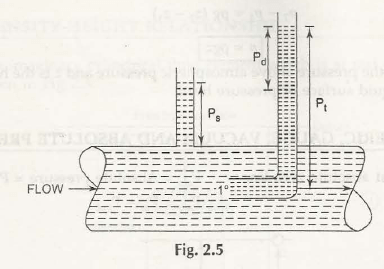Static Pressure is the pressure of fluid on the body or wall of the system and total pressure is the summation of all pressures. These can be determined for different fluid flow situations as shown in Fig. 2.5.Let us consider the following to measure the value of static and total pressure:

• To calculate the static pressure, a vertical tube is fitted to the wall of pipe. The pressure is measured by the raising of water level i.e., Ps
• To calculate the total pressure, a pitot tube should be fitted to the wall of pipe. The difference between Pt and Pd shows the total pressure of fluid.

Example 2.1:

A hydraulic press is used to lift 30 KN loads whose plunger diameter is 10 cm and stroke length is 40 cm. If it can execute up to 120 strokes per minute with its ram of diameter 40cm, find out the force required at plunger. Also, find the power required to drive this plunger and the distance to raise load.

Solution:

Given Data:

Pressure on plunger, P1 = f/ a

Pressure on ram, P2 = W/ A

We know that,

P1 =P2

f/ a = W/ A

Or,

f = W . a/ A

Substituting all values, we get-

f = 1875 N

Now,

Stroke volume on plunger = 0.4 x/ 4 x 0.12

Stroke volume on ram = Sr x / 4 x 0.42

We know that,

Stroke volume on plunger = Stroke volume on ram

0.4 x/ 4 x 0.12 = Sr x / 4 x 0.42

Or,

Sr = 0.025 m

So, distance raised can be given by-

0.025 x 120 = 3 m/ min

And,

Power  = 30 x 103 x 3 / 60

= 1.5 kW

Example 2.2

Find the height of liquid when the pressure at a point is exerted up to 4 N/cm2. Take liquid as water and oil (specific gravity = 0.8).

Solution:

We know that,

Height, z = / pg

• For Water:

z = 4 x 104/ 1000 x 9.81

= 4.08 m

• For Oil:

z = 4 x 104/ 0.8 x 1000 x 9.81

= 5.1 m

Example 2.3:

Height of oil from a certain point is 50m. If the specific gravity is 0.8, find its corresponding water head.

Solution:

We know that,

Height, z = / pg

= 0.8 x 1000 x 9.81 x 50/ 1000 x 9.81

= 40 m

Example 2.4:

A hydraulic jack of diameters 2 cm and 8 cm is exerted by a force of 100 N. Determine the load lifted by its piston, when:

• Both pistons are at same level
• Small piston is kept at 50 cm above its large piston

Solution:

From the given data, we can find the solution as-

• Both pistons are at same level

Pressure, P = 323215 N/m2

• Small piston is kept at 50 cm above its large piston

W = P x A

= 323215 x / 4 (0.08)2

= 1624.6 N

Links of Previous Main Topic:-

Links of Next Mechanical Engineering Topics:-

### Submit Your Assignment### Customer Reviews

My Homework Help
Rated 5.0 out of 5 based on 510 customer reviews at